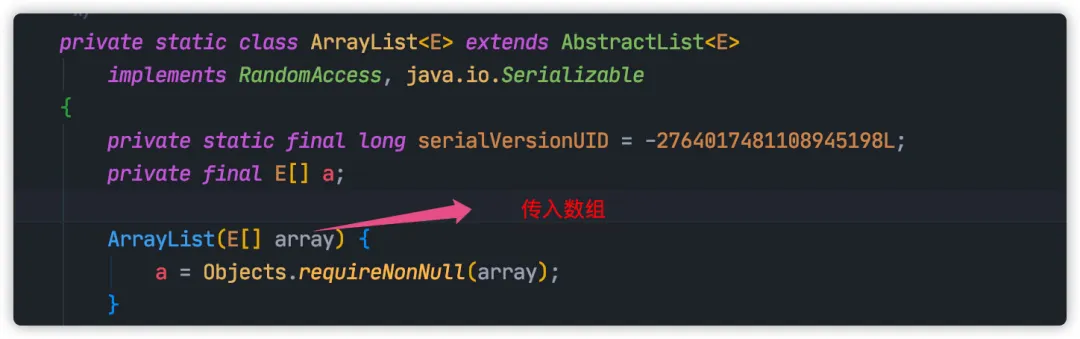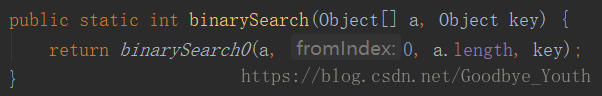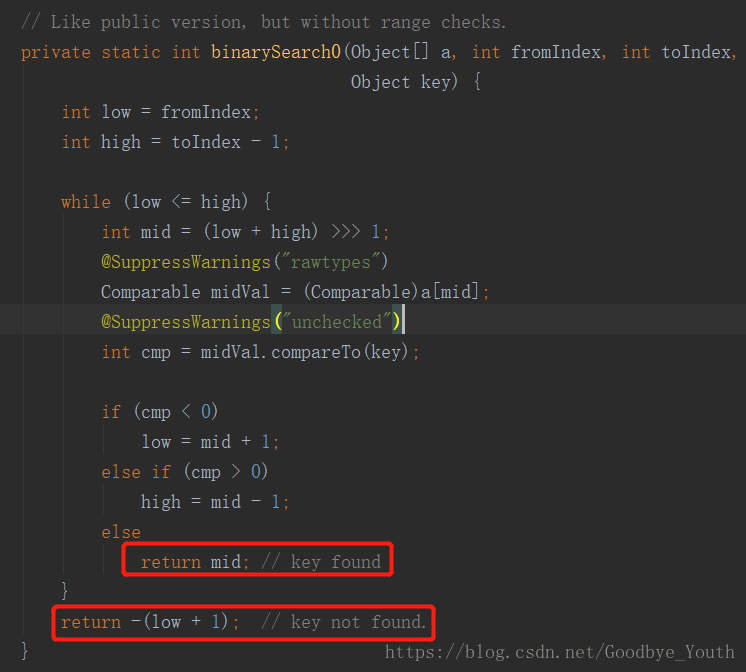• //导入Java中util类中的Arrays工具包
千次阅读
2021-03-14 00:49:15

源自：7-1 编程练习

//导入Java中util类中的Arrays工具包

//导入Java中util类中的Arrays工具包

import java.util.Arrays;

//创建一个HelloWorld类

public class HelloWorld {

//导入一个 main 方法

public static void main(String[] args) {

//创建一个对象：hello

HelloWorld hello = new HelloWorld();

//定义数组变量并赋值

int[] scores = {89,-23,64,91,119,52,73};

//调用方法并传入成绩信息

hello.sort(scores);

//输出"考试成绩的前三名为："

System.out.println("考试成绩的前三名为：");

//定义方法完成成绩排序并输出前三名的功能

public int sort(int[] scores){

Arrays.sort(scores);

int num=0;

int nuy=0

for ( int i=scores.length-1;i>=0;i--) {

if( scores[i]>100){

coutiune;

}

num++;

if(num>3){

break;

}

System.out.println(scores[i]);

}

}

}

提问者：weixin_二个工人_0

2018-03-20 21:31

更多相关内容
• 关于Arrays类的使用及方法： Arrays是静态的数组：存在于util包里面，需导入；常用方法： Object copyOf(Object a[], int newLength); 复制数组 Object copyOfRange(Object a[], int from ,int to); 复制数组从from...

## 关于Arrays类的使用及方法：

Arrays是静态的数组：存在于util包里面，需导入；常用方法：

1. Object copyOf(Object a[], int newLength); 复制数组
2. Object copyOfRange(Object a[], int from ,int to); 复制数组从from到to
3. Arrays.equals(Object a [] , Object b []); 比较数组（同样类型）
4. void fill (Object a[] , object); 将object元素值分配给指定Object 型数组指定范围中的每个元素
5. Arrays.toString(Object a []);返回指定数组内容的字符串表示形式
6. void sort(Object[] a) ; 根据元素的自然顺序对指定对象数组按升序进行排序
7. int binarySearch(Object [] a, Object key)； 使用二分搜索法来搜索指定的Object型数组的范围，以获得指定的关键字的索引。
(具体其他详查API)

public class Main {
public static void main(String[] args) {
int a[] = new int[]{1,8,9,7,4,5,6,0};
int b[] = new int;
int c[] = new int;
int [] d = Arrays.copyOfRange(a,0,5);
int i =0;
b = Arrays.copyOf(a,8);
Arrays.fill(c,8);
Arrays.sort(b);
System.out.print("使用copyOf()方法复制数组：");
for (;i<b.length;i++){
System.out.print(b[i]+" ");
}

展开全文java
• 本文用示例介绍Java中的Arrays的常用方法。 Arrays用来操作数组，比如：拷贝数组、转换为list、填充等。 asList 数组/多个类都可以 package org.example.a; import java.util.Arrays; import java.util.List...

# 简介

本文用示例介绍Java中的Arrays的常用方法。

Java中的Arrays是用来操作数组的工具类，支持如下功能：拷贝数组、转换为list、填充等。

# asList

数组/多个类都可以

package org.example.a;

import java.util.Arrays;
import java.util.List;

public class Demo {
public static void main(String[] args) throws ClassNotFoundException {
String s1 = "abcd";
String s2 = "efg";
String[] strings = {"hi", "jk", "lm"};
List<String> list1 = Arrays.asList(s1, s2);
List<String> list2 = Arrays.asList(strings);
//下边这样就不可以了
//List<String> list3 = Arrays.asList(s1, s2, strings);

System.out.println(list1);
System.out.println(list2);
}
}

执行结果

[abcd, efg]
[hi, jk, lm]

基础数组会作为一个元素

package org.example.a;

import java.util.Arrays;
import java.util.List;

public class Demo {
public static void main(String[] args) throws ClassNotFoundException {
int[] a = {1, 2, 3};
List<int[]> list = Arrays.asList(a);

System.out.println(Arrays.toString(list.get(0)));
}
}

执行结果

[1, 2, 3]

不支持增删，元素共享

Arrays$ArrayList：不支持增删操作；共享原始数据（Arrays$ArrayList元素与Arrays的数组元素是共享的）。

源码（增加）

package org.example.a;

import java.util.Arrays;
import java.util.List;

public class Demo {
public static void main(String[] args) {
String[] strings = {"1", "2", "3"};
List<String> list = Arrays.asList(strings);
System.out.println(list);
}
}

运行结果

at org.example.a.Demo.main(Demo.java:10)

分析

Arrays#asList 返回的 ArrayList 只是 Arrays 一个内部类，并非真正的 java.util.ArrayList。

java.util.ArrayList和Arrays$ArrayList都继承自 AbstractList。而 java.util.Arrays$ArrayList 并没有重写父类的add/remove方法。而父类方法恰恰都会抛出 UnsupportedOperationException。

源码（修改）

package org.example.a;

import java.util.Arrays;
import java.util.List;

public class Demo {
public static void main(String[] args) {
String[] strings = {"1", "2", "3"};
List<String> list = Arrays.asList(strings);
list.set(0, "modify_1");
strings = "modify_2";
System.out.println(list);
}
}

执行结果

[modify_1, modify_2, 3]

原因

查看 java.util.Arrays\$ArrayList 实现，我们可以发现底层实际使用了原始数组。解决方法

法1：外边套一层ArrayList

List<String> list = new ArrayList<>(Arrays.asList(arrays));

法2：使用谷歌的Guava包的Lists.newArrayList

List<String> list = Lists.newArrayList(arrays);

# binarySearch

Arrays.binarySearch(Object[] array, Object key)

注意：在调用该方法之前，必须先调用 Arrays.sort() 方法进行排序，如果数组没有排序，那么结果是不确定的，此外如果数组中包含多个指定元素，则无法保证将找到哪个元素

使用二分法查找数组内指定元素的索引值

当搜索元素是数组元素时，返回该元素的索引值
当搜索元素不是数组元素时，返回 - (索引值 + 1)

搜索元素是数组元素，返回该元素索引值

Integer[] data = {1, 3, 5, 7};
Arrays.sort(data);
System.out.println(Arrays.binarySearch(data, 1)); // 0


搜索元素不是数组元素，且小于数组中的最小值

Integer[] data = {1, 3, 5, 7};
Arrays.sort(data);
// 此时程序会把数组看作 {0, 1, 3, 5, 7}，此时0的索引值为0，则搜索0时返回 -(0 + 1) = -1
System.out.println(Arrays.binarySearch(data, 0)); // -1


搜索元素不是数组元素，且大于数组中的最大值

Integer[] data = {1, 3, 5, 7};
Arrays.sort(data);
// 此时程序会把数组看作 {1, 3, 5, 7， 9}，此时9的索引值为4，则搜索8时返回 -(4 + 1) = -5
System.out.println(Arrays.binarySearch(data, 9)); // -5


搜索元素不是数组元素，但在数组范围内

Integer[] data = {1, 3, 5, 7};
Arrays.sort(data);
// 此时程序会把数组看作 {1, 2, 3, 5, 7}，此时2的索引值为1，则搜索2时返回 -(1 + 1) = -2
System.out.println(Arrays.binarySearch(data, 2)); // -2


Arrays.binarySearch(Object[] array, int fromIndex, int toIndex, Object obj)

使用二分法查找数组内指定范围内的指定元素的索引值

Integer[] data = {1, 3, 5, 7};
Arrays.sort(data);
// {1, 3}，3的索引值为1
System.out.println(Arrays.binarySearch(data, 0, 2, 3)); // 1


看下 binarySearch() 方法的源码，对了解该方法有很大的帮助从源码中可以看到

• 当搜索元素是数组元素时，返回该元素的索引值
• 当搜索元素不是数组元素时，返回 - (索引值 + 1)

# copyOf

Arrays.copyOf(T[] original, int newLength)

拷贝数组，其内部调用了 System.arraycopy() 方法，从下标 0 开始，如果超过原数组长度，则会用 null 进行填充

Integer[] data1 = {1, 2, 3, 4};
Integer[] data2 = Arrays.copyOf(data1, 2);
System.out.println(Arrays.toString(data2)); // [1, 2]
Integer[] data3 = Arrays.copyOf(data1, 5);
System.out.println(Arrays.toString(data3)); // [1, 2, 3, 4, null]


Arrays.copyOfRange(T[] original, int from, int to)

拷贝数组，指定起始位置和结束位置，如果超过原数组长度，则会用 null 进行填充

Integer[] data1 = {1, 2, 3, 4};
Integer[] data2 = Arrays.copyOfRange(data1, 0, 2);
System.out.println(Arrays.toString(data2)); // [1, 2]
Integer[] data2 = Arrays.copyOfRange(data1, 0, 5);
System.out.println(Arrays.toString(data2)); // [1, 2, 3, 4, null]


# equals

Arrays.equals(Object[] array1, Object[] array2)

判断两个数组是否相等（如果两个数组被认为是相等的，则两个数组中应包含相同顺序的相同元素）

• 数组元素为基本数据类型时，依次比较值
• 数组元素为引用数据类型时，依次调用元素的 equals() 方法进行比较
Integer[] data1 = {1, 2, 3};
Integer[] data2 = {1, 2, 3};
System.out.println(Arrays.equals(data1, data2)); // true


Arrays.deepEquals(Object[] array1, Object[] array2)

判断两个多维数组是否相等（如果两个多维数组被认为是相等的，则两个数组中应包含相同顺序的相同元素）

• 数组元素为基本数据类型时，依次比较值
• 数组元素为引用数据类型时，依次调用元素的 equals() 方法进行比较
Integer[][] data1 = {{1,2,3}, {1,2,3}};
Integer[][] data2 = {{1,2,3}, {1,2,3}};
System.out.println(Arrays.deepEquals(data1, data2)); // true


# fill

Arrays.fill(Object[] array, Object obj)

用指定元素填充整个数组 (会替换掉数组中原来的元素)

Integer[] data = {1, 2, 3, 4};
Arrays.fill(data, 9);
System.out.println(Arrays.toString(data)); // [9, 9, 9, 9]

Arrays.fill(Object[] array, int fromIndex, int toIndex, Object obj)

用指定元素填充数组，从起始位置到结束位置，取头不取尾 (会替换掉数组中原来的元素)

Integer[] data = {1, 2, 3, 4};
Arrays.fill(data, 0, 2, 9);
System.out.println(Arrays.toString(data)); // [9, 9, 3, 4]

# hashCode

Arrays.hashCode(Object[] array)

返回数组的哈希值

Integer[] data = {1, 2, 3};
System.out.println(Arrays.hashCode(data)); // 30817


Arrays.deepHashCode(Object[] array)

返回多维数组的哈希值

Integer[][] data = {{1, 2, 3}, {1, 2, 3}};
System.out.println(Arrays.deepHashCode(data)); // 987105


# parallelPrefix

Arrays.parallelPrefix(T[] array, BinaryOperator op)

让数组中的所有元素，并行地使用方法提供的生成器函数来计算每个元素 (二元操作)，当数据规模较大时，会有更好的性能

Integer[] data = {2, 3, 4, 5};
// 第一个元素2不变，将其与第二个元素3一起作为参数x, y传入，得到乘积6，作为数组新的第二个元素
// 再将6和第三个元素4一起作为参数x, y传入，得到乘积24，作为数组新的第三个元素，以此类推
Arrays.parallelPrefix(data, (x, y) -> x * y);
System.out.println(Arrays.toString(data)); // [2, 6, 24, 120]


Arrays.parallelPrefix(T[] array, int fromIndex, int toIndex, BinaryOperator op)

让指定范围内的数组元素，并行地使用方法提供的生成器函数来计算每个元素 (二元操作)，当数据规模较大时，会有更好的性能

Integer[] data = {2, 3, 4, 5};
// 第一个元素2不变，将其与第二个元素3一起作为参数x, y传入，得到乘积6，作为数组新的第二个元素
// 再将6和第三个元素4一起作为参数x, y传入，得到乘积24，作为数组新的第三个元素，以此类推
Arrays.parallelPrefix(data, 0, 3, (x, y) -> x * y);
System.out.println(Arrays.toString(data)); // [2, 6, 24, 5]


# setAll

Arrays.setAll(T[] array, IntFunction<? extends T> generator)

让数组中的所有元素，串行地使用方法提供的生成器函数来计算每个元素 (一元操作)

Integer[] data = {1, 2, 3, 4};
// i为索引值
Arrays.setAll(data, i -> data[i] * 2);
System.out.println(Arrays.toString(data)); // [2, 4, 6, 8]


Arrays.parallelSetAll(T[] array, IntFunction<? extends T> generator)

让数组中的所有元素，并行地使用方法提供的生成器函数来计算每个元素 (一元操作)，当数据规模较大时，会有更好的性能

Integer[] data = {1, 2, 3, 4};
// i为索引值
Arrays.parallelSetAll(data, i -> data[i] * 2);
System.out.println(Arrays.toString(data)); // [2, 4, 6, 8]


# spliterator

Arrays.spliterator(T[] array)

返回数组的分片迭代器，用于并行地遍历数组

public class Students {

private String name;

private Integer age;

public Students(String name, Integer age) {
this.name = name;
this.age = age;
}
// 省略get、set方法
}

public static void main(String[] args) {
Students[] data = new Students;
IntStream.range(0,5).forEach(i -> data[i] = new Students("小明"+i+"号", i));
// 返回分片迭代器
Spliterator<Students> spliterator = Arrays.spliterator(data);
spliterator.forEachRemaining(stu -> {
System.out.println("学生姓名: " + stu.getName() + "  " + "学生年龄: " + stu.getAge());
});
}


运行结果

学生姓名: 小明0号  学生年龄: 0
学生姓名: 小明1号  学生年龄: 1
学生姓名: 小明2号  学生年龄: 2
学生姓名: 小明3号  学生年龄: 3
学生姓名: 小明4号  学生年龄: 4

# sort

Arrays.sort(Object[] array)

对数组元素进行排序 (串行排序)

String[] data = {"1", "4", "3", "2"};
System.out.println(Arrays.toString(data)); // [1, 4, 3, 2]
Arrays.sort(data);
System.out.println(Arrays.toString(data)); // [1, 2, 3, 4]

Arrays.sort(T[] array, Comparator<? super T> comparator)

使用自定义比较器，对数组元素进行排序 (串行排序)

String[] data = {"1", "4", "3", "2"};
System.out.println(Arrays.toString(data)); // [1, 4, 3, 2]
// 实现降序排序，返回-1放左边，1放右边，0保持不变
Arrays.sort(data, (str1, str2) -> {
if (str1.compareTo(str2) > 0) {
return -1;
} else {
return 1;
}
});
System.out.println(Arrays.toString(data)); // [4, 3, 2, 1]

Arrays.sort(Object[] array, int fromIndex, int toIndex)

对指定范围内的数组元素进行排序 (串行排序)

String[] data = {"1", "4", "3", "2"};
System.out.println(Arrays.toString(data)); // [1, 4, 3, 2]
// 对下标[0, 3)的元素进行排序，即对1，4，3进行排序，2保持不变
Arrays.sort(data, 0, 3);
System.out.println(Arrays.toString(data)); // [1, 3, 4, 2]

Arrays.sort(T[] array, int fromIndex, int toIndex, Comparator<? super T> c)

使用自定义比较器，对指定范围内的数组元素进行排序 (串行排序)

String[] data = {"1", "4", "3", "2"};
System.out.println(Arrays.toString(data)); // [1, 4, 3, 2]
// 对下标[0, 3)的元素进行降序排序，即对1，4，3进行降序排序，2保持不变
Arrays.sort(data, 0, 3, (str1, str2) -> {
if (str1.compareTo(str2) > 0) {
return -1;
} else {
return 1;
}
});
System.out.println(Arrays.toString(data)); // [4, 3, 1, 2]

Arrays.parallelSort(T[] array)

注意：其余重载方法与 Arrays.sort() 相同

对数组元素进行排序 (并行排序)，当数据规模较大时，会有更好的性能

String[] data = {"1", "4", "3", "2"};
Arrays.parallelSort(data);
System.out.println(Arrays.toString(data)); // [1, 2, 3, 4]


# toString

Arrays.toString(Object[] array)

返回数组元素的字符串形式

Integer[] data = {1, 2, 3};
System.out.println(Arrays.toString(data)); // [1, 2, 3]


Arrays.deepToString(Object[] array)

返回多维数组元素的字符串形式

Integer[][] data = {{1, 2, 3}, {1, 2, 3}};
System.out.println(Arrays.deepToString(data)); // [[1, 2, 3], [1, 2, 3]]


# stream

Arrays.stream(T[] array)

返回数组的流 (Stream)，然后我们就可以使用 Stream 相关的许多方法了

Integer[] data = {1, 2, 3, 4};
List<Integer> list = Arrays.stream(data).collect(toList());
System.out.println(list); // [1, 2, 3, 4]

# 其他网址

Class -- 02 -- Arrays类常用方法解析_Gene Xu-CSDN博客_arrays类

展开全文java
• Arrays 类是一个工具类，其中包含了数组操作的很多方法。这个 Arrays 类里均为 static 修饰的方法(static 修饰的方法可以直接通过类名调用)，可以直接通过 Arrays.xxx(xxx) 的形式调用方法。1)int binarySearch(type...

Arrays 类是一个工具类，其中包含了数组操作的很多方法。这个 Arrays 类里均为 static 修饰的方法(static 修饰的方法可以直接通过类名调用)，可以直接通过 Arrays.xxx(xxx) 的形式调用方法。

1)int binarySearch(type[] a, type key)

使用二分法查询 key 元素值在 a 数组中出现的索引，如果 a 数组不包含 key 元素值，则返回负数。调用该方法时要求数组中元素己经按升序排列，这样才能得到正确结果。

2)int binarySearch(type[] a, int fromIndex, int toIndex, type key)

这个方法与前一个方法类似，但它只搜索 a 数组中 fromIndex 到 toIndex 索引的元素。调用该方法时要求数组中元素己经按升序排列，这样才能得到正确结果。

3)type[] copyOf(type[] original, int length)

这个方法将会把 original 数组复制成一个新数组，其中 length 是新数组的长度。如果 length 小于 original 数组的长度，则新数组就是原数组的前面 length 个元素，如果 length 大于 original 数组的长度，则新数组的前面元索就是原数组的所有元素，后面补充 0(数值类型)、false(布尔类型)或者 null(引用类型)。

4)type[] copyOfRange(type[] original, int from, int to)

这个方法与前面方法相似，但这个方法只复制 original 数组的 from 索引到 to 索引的元素。

5)boolean equals(type[] a, type[] a2)

如果 a 数组和 a2 数组的长度相等，而且 a 数组和 a2 数组的数组元素也一一相同，该方法将返回 true。

6)void fill(type[] a, type val)

该方法将会把 a 数组的所有元素都赋值为 val。

7)void fill(type[] a, int fromIndex, int toIndex, type val)

该方法与前一个方法的作用相同，区别只是该方法仅仅将 a 数组的 fromIndex 到 toIndex 索引的数组元素赋值为 val。

8)void sort(type[] a)

该方法对 a 数组的数组元素进行排序。

9)void sort(type[] a, int fromIndex, int toIndex)

该方法与前一个方法相似，区别是该方法仅仅对 fromIndex 到 toIndex 索引的元素进行排序。

10)String toString(type[] a)

该方法将一个数组转换成一个字符串。该方法按顺序把多个数组元素连缀在一起，多个数组元素使用英文逗号,和空格隔开。

展开全文java数组类的调用
• 本文将会简单介绍Arrays类的使用方法，代码如下： package com.xzw.csdn; import java.util.Arrays; /** * Arrays类的应用 * @author xzw * */ public class UseOfArrays { /** * 主函数 * @param ...java
• ## Java中的Arrays类使用详解

万次阅读 多人点赞 2017-04-27 11:28:21
分析：如果是arr1.equals(arr2),则返回false，因为equals比较的是两个对象的地址，不是里面的数，而Arrays.equals重写了equals，所以，这里能比较元素是否相等。 这里补充一下，如果还是不用Arrays.equals，那么...java class
• 1、定义：Arrays类是一系列对数组进行操作方法； 2、使用 1.导入包：import java.util.Arrays 2.使用函数 （1）Arrays.toString(Object []array) //返回数组的字符串形式 例子： public class Arrays_...java 算法
• 另一种方式是使用Comparator接口：编写多个排序方式类实现Comparator接口，并重写新Comparator接口中的compare()方法，在调用Arrays的sort()时将排序类对象作为参数传入：public static <T> void sort(T[] a,...java Comparable Comparator
• Arrays工具类asList（）方法以及实例引入集合嵌套问题1.Arrays工具类的asList()方法的使用1.1 Arrays工具类中asList()方法传入基本数据类型的数组1.2 Arrays工具类中asList()方法传入一个引用数据类型的数组1.3 ...java
• //输出内存地址 Arrays类 头文件添加： import java.util.Arrays; toString方法 int[] array = {1, 4, 2, 5, 23, 56}; System.out.println(array); //输出[I@1b6d3586 System.out.println(Arrays.toString(array));...java
• 在网上发现一篇讲解 Arrays.asList 用法的好文章：Java Array to List Examples，我把文章要点整理如下，并加上一些个人见解，恳请各位看官斧正。一、java.util.Arrays.asList() 的一般用法List 是一种很有用的数据...
• 数组Arrays1. 数组复制1.1 概述1.2 用法1.3 程序范例1.3.1 利用System.arraycopy实现1.3.2 利用copyOfRange实现2. 数组转换为字符串2.1 概述2.2 程序范例3. 数组排序3.1 概述3.2 程序范例4. 数组元素查找4.1 概述4.2...
• arrays.sort(. 我们都使用Arrays.sort对对象和原始数组进行排序。 此API在下面使用合并排序或Tim排序对内容进行排序，如下所示： public static void sort(Object[] a) { if (LegacyMergeSort.userRequested) ...
• 我们都使用Arrays.sort对对象和原始数组进行排序。 该API在下面使用合并排序或Tim排序对内容进行排序，如下所示： public static void sort(Object[] a) { if (LegacyMergeSort.userRequested) legacyMergeSort(a...
• 文章目录1、Arrays.sort(int[] a)2、Arrays.sort(int[] a, int fromIndex, int toIndex)3、public static void sort(T[] a,int fromIndex,int toIndex, Comparator<? super T> c)二维数组定制排序： 1、Arrays...java 数据结构
• 1 导入 java.util.Arrays 包。 2 使用 Armys.sort(数组名) 语法对数组进行排序，排序规则是从小到大，即升序。 默认：升序 import java.util.Arrays; public class Test1 { public static void main(String[] args)...java
• 在给一个数组冒泡排序，最后输出的时候用了Arrays.toString(XXX)方法，结果出错了，导入了包也不能解决。后来终于解决了，只是很小的一个错误点。 Arrays.toString(XXX)方法是数组工具类Arrays提供的，用于将数组...字符串 java
• list = Arrays.asList("a","b","c"); 但你可能不知道这样得到的List它的长度是不能改变的。当你向这个List添加或删除一个元素时（例如 list.add("d");）程序就会抛出异常（java.lang.UnsupportedOperat...
• def runplt(): plt.figure() plt.title(u’低氧环境下运动员白细胞含量随...TypeError: only size-1 arrays can be converted to Python scalars 我只是把y改成这种形式以后才出现的这个问题，请问如何解决？
• 问题引入 在我们需要向一个方法中传递List的时候，经常会这样写： List<String> list = new ArrayList<String>(); list.add("工具类"); list.add("计算机类"); book.setType(list); 其实这四行直接...list java
• Java Arrays class consists exclusively of static methods that operates on array. Java Arrays类仅由对数组进行操作的静态方法组成。 Java数组 (Java Arrays) Java Arrays class was introduced in Java 1.2 ...java python 编程语言 数据结构
• Arrays.sort()分析 基本类型 对于基本类型，Java7前使用插入排序与快速排序，Java7之后引用双轴快速排序(DualPivotQuicksort)代替快速排序 当数组中元素小于7时，采用插入排序，尽管插入排序的时间复杂度为平方级，...
• 一、java.util.Arrays.asList() 的一般用法 如果将一个数组转换为 List，可以将需要转化的数组作为参数，或者直接把数组元素作为参数。但是其中参数必须是包装类类型，如果使用的是基本数据类型，可以使用Jdk8新...
• ## Java:import java.util.Arrays(一)的使用

千次阅读 多人点赞 2020-05-25 21:39:44
Arrays.sort() (从小到大排序（即升序）) 结果： 2.Arrays.binarySerch()：找到定位数组的下标： 结果： Arrays.binarySerch()出现负数（-2）的原因是：binarySearch()方法的返回值为： 1、如果找到关键字，则...java
• class Test{ public static void main(String[] args) { int[] nums={4,5,7,8,0,3}; Integer[] numsArr = new Integer[nums.length]; for (int i = 0; i < nums.length; i++) { ... Arrays..java
• Arrays.asList()这个方法可以将数组转换为集合。1.List list = Arrays.asList(reports);String reportStr= "皕杰报表,分析报表,部署报表";String[] reports = reportStr.split(",");List list = Arrays.asList...
• ## Arrays.asList使用简介

千次阅读 2019-07-15 15:23:34
一、java.util.Arrays.asList() 的一般用法   List 是一种很有用的数据结构，如果需要将一个数组转换为 List 以便进行更丰富的操作的话，可以这么实现： String[] myArray = { "Apple", "Banana", "Orange" }；...
• Arrays属于rt.jar中java.util包下面的类，不需要引入其他外部jar,而ArrayUtils属于commons-lang.jar中org.apache.commons.lang3包下下面的类，要想使用此类需要引入包。 一.Arrays方法介绍 1.asList方法，很方便的......